# Relating Airy and Bessel functions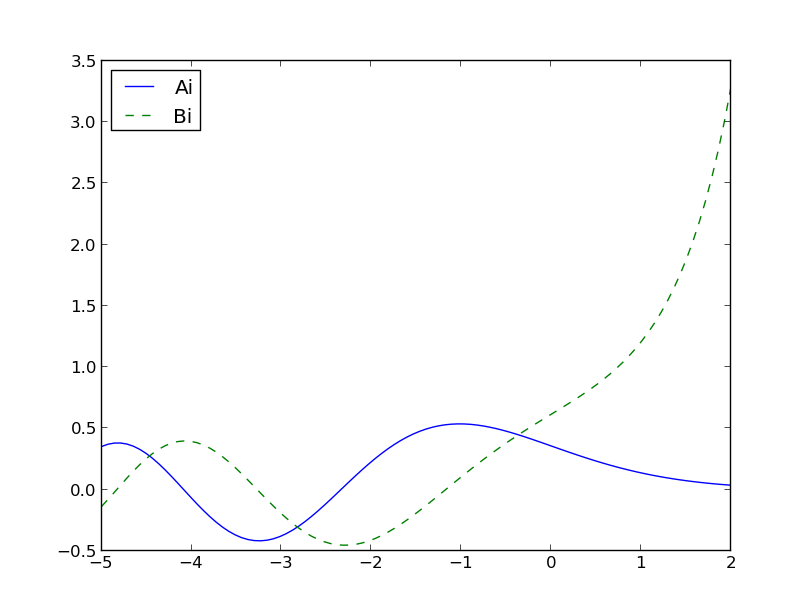Reblogged from The Endeavour by J. D. Cook.

The Airy functions Ai(x) and Bi(x) are independent solutions to the differential equation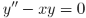For negative x they act something like sin(x) and cos(x). For positive x they act something like exp(x) and exp(-x). This isn’t surprising if you look at the differential equation. If you replace xwith a negative constant, you sines and cosines, and if you replace it with a positive constant, you get positive and negative exponentials.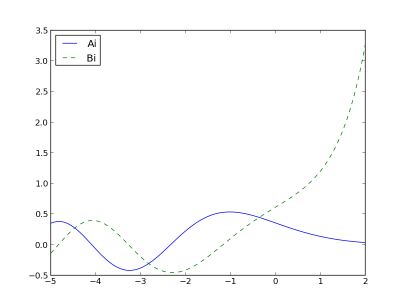The Airy functions can be related to Bessel functions as follows: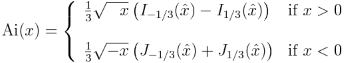and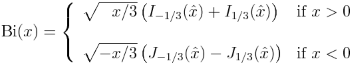Here J is a “Bessel function of the first kind” and I is a “modified Bessel function of the first kind.” Also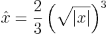To verify the equations above, and to show how to compute these functions in Python, here’s some code.

The SciPy function `airy` computes both functions, and their first derivatives, at once. I assume that’s because it doesn’t take much longer to compute all four functions than to compute one. The code for `Ai2` and `Bi2` below uses `np.where` instead of `if... else` so that it can operate on NumPy vectors all at once. You can plot `Ai` and  `Ai2` and see that the two curves lie on top of each other. The same holds for `Bi` and  `Bi2` .

```from scipy.special import airy, jv, iv
from numpy import sqrt, where

def Ai(x):
(ai, ai_prime, bi, bi_prime) = airy(x)
return ai

def Bi(x):
(ai, ai_prime, bi, bi_prime) = airy(x)
return bi

def Ai2(x):
third = 1.0/3.0
hatx = 2*third*(abs(x))**1.5
return where(x > 0,
third*sqrt( x)*(iv(-third, hatx) - iv(third, hatx)),
third*sqrt(-x)*(jv(-third, hatx) + jv(third, hatx)))

def Bi2(x):
third = 1.0/3.0
hatx = 2*third*(abs(x))**1.5
return where(x > 0,
sqrt( x/3.0)*(iv(-third, hatx) + iv(third, hatx)),
sqrt(-x/3.0)*(jv(-third, hatx) - jv(third, hatx)))```

There is a problem with `Ai2` and `Bi2`: they return `nan` at 0. A more careful implementation would avoid this problem, but that’s not necessary since these functions are only for illustration. In practice, you’d simply use `airy` and it does the right thing at 0.

This site uses Akismet to reduce spam. Learn how your comment data is processed.